# ESSENTIAL EQUATIONS OF TURBOMACHINES

Discussion about Euler equation ● Relation between mean aerodynamic velocity and force F ● Force on blade and circulation of velocity
The article of online continued resource Transformační technologie; ISSN 1804-8293;
www.transformacni-technologie.cz; Copyright©Jiří Škorpík, 2006-2020. All rights reserved. This work was published without any linguistic and editorial revisions..

## Introduction

Terms as force on blades, torque, work (power) can be explained easily and intuitively through a description function of a water wheel:

What dimensions must have a water wheel on a weir about height 0,6 m and at mass flow rate 0,7 m3·s-1? For calculation will be used empirical knowledges of millwrights and millers . The solution of this problem is shown in the Appendix 255.
Problem 1.255Figure at Problem 1.
d [m] wheel diameter; a [m] blade height; A [m2] flow area; c [m·s-1] absolute velocity of water;

The information about the force on blades, the torque, the schaft speed, and the power are significant for all types of turbomachines. For calculation of these quantities can be derived general equations, which may be used for all type of turbomachines (history of the derivation Essential equations of turbomachines are shown in the chapter 1. Water wheels and water turbines).

## Force on blades from fluid stream

The velocity and the direction of the working fluid stream are changed inside the turbomachine stage. It means that some force acts to the working fluid. This force is function of mass flow rate and shape of volume through which working fluid flows (a control volume):

● 1 ●
● 12. Essential equations of turbomachines ●1.196 Force on blades from fluid stream.
Velocities and forces are vectors, but the arrow above the symbol does not usually write. H [N] momentum of working fluid; Fh [N] weight forces, which act on working fluid inside control volume (gravitational force); Fp [N] pressure forces, which act on working fluid on border of control volume; Ft [N] force acting on working fluid from bodies inside or on border of control volume (blades, casing etc.); F [N] force acting on bodies inside or on border of control volume (blades, casing atc.) from working fluid; w [m·s-1] relative velocity; u [m·s-1] tangential velocity; m [kg·s-1] mass flow rate of working fluid which flows through control volume; m [m] weight of working fluid inside control volume; p [Pa] pressure; A [m2] surface of control volume; g [m·s-2] gravitational acceleration. Ψ streamline of absolute velocity, K control volume; 1 inlet to control volume; 2 exit from control volume. This equation is called Euler equation, because it was derived by Leonhard Euler as first. This equation agrees for stationary flow through the control volume. The derivation of the Euler equation is shown in the Appendix 196.

The control volume for the case of Figure 1b is defined so way: working fluid has the inlet to the control volume through the boundary AB (flow area A1) and outlets from the control volume through the boundary CD (flow area A2). The boundaries of the control volumes AD and BC are the same streamlines, therefore the pressure force here are the same but opposite between himsel. The control volume is not moved.

For calculation of force on blades, according Euler equation, are sufficient parameters on boundary of the control volume.

The force F has three spatial components as absolute velocity: at axial direction Fa (this components of the force causes a stress of rotor shaft at axial direction, this force acts to axial bearing of shaft), at radial direction Fr, at tangential direction Fu (this force does torque on rotor shaft):

Some components of force F can be negligible (e.g.: for case of the axial stage of turbomachine there is not radial component – flow on surface of a cylinder surface; for case of radial stage of a fan there is not axial component etc.).

● 2 ●
● 12. Essential equations of turbomachines ●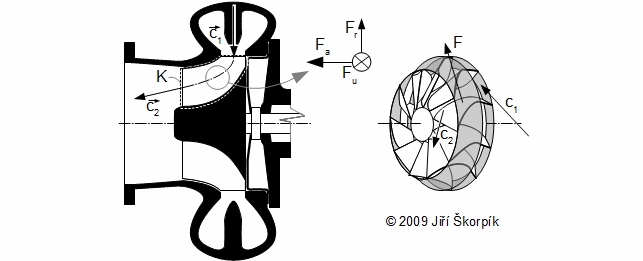2.274 An illustration of force on blades of radial turbine with axial exit.
At right from sectional view is 3D view of the impeller.
What force on blades of water turbine (axial type)? It is known dimension of the rotor, the flow mass rate, the shaft speed and the velocities of working gas. The solution of this problem is shown in the Appendix 583.
Problem 2.583

The boundaries of control volume AD and BC inside blade row may not copy streamlines of absolute velocity, but suffice it to define so that flow parameters at these boundaries were the same. Boundaries of the control volume are usually defined by streamlines of relative velocities for cases of turbomachines. For this type the control volume is momentum of the stream on boundary AD the same as on boundary BC but opposite direction, therefore these momentum are eliminated between themselves:3.256 The force on the blade of the axial turbine stage (u1=u2 – outlet of the working fluid from stage is on the same diameter as at inlet).
s [m] pitch of blade row. For the case of the axial stage does not arise radial force on blades Fr=0.
Water flows through of the pipe with velocity 4 m·s-1. What is force on the pipe between the flanges from flow of liquid? Inside diameter of the pipe is 23 mm, difference of height between the upper and bottom flange is 1,2 m, difference between pressure inside pipe and atmospheric pressure is 2 m water column. Ideal fluid and flow without losses. The solution of this problem is shown in the Appendix 254.
Problem 3.254
● 3 ●
● 12. Essential equations of turbomachines ●Figure at Problem 3.
pat [Pa] atmospheric pressure.

The force F can be computed from relative flow through Bernoulli equation for a rotating passage [1, p. 41], but this caculation is complicated – (in this case is computed with Coriolis force which has influence force to tangential direction, and centrifugal force which has influence force to radial direction):

Describe forces inside blade passage of radial impeller of fan from fluid stream, if its relative velocities are known and its geometry. Inlet and outlet pressure are same p1=p2. The solution of this problem is shown in the Appendix 584.
Problem 4.584

The force from pressure Fp acts to the control volume. If the inlet flow area (to blade passages) A1 is the same as exit flow area A2, then for a case of ρ=const., is true w1=w2 and p1=p2. This turbomachine stage is called impulse stage. The force F is the same as tangential force Fu(1) for cases of impulse stages with negligible weight forces Fh and losses: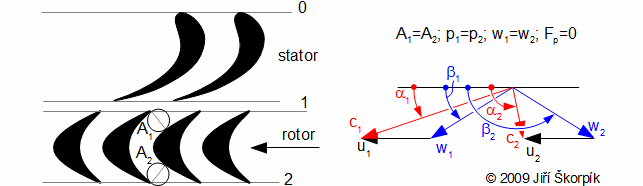4.279 An example of the impulse stage (this stage is used by Laval turbine).
● 4 ●
● 12. Essential equations of turbomachines ●
(1)Remark
This is true for flow without losses. In other way does not have to be truth if A1=A2 then p1=p2 (the losses increase specific volume of working gas see article 37. Throttling of gases and steam).

Radial stages can be designed such as an impulse stage(2):ω [rad·s-1] angular velocity; r [m] impeller. The picture shows an example of radial stage with forward blades. For case working machine stage is the direction of the tangential force Fu opposite to the direction of tangential velocity (work enters to the working fluid).
(2)Remark
It is true at negligible centrifugal force inside working fluid volume between radius r1=r2, otherwise w2>w1 see Problem 4.

If the inlet flow area A1 is not the same as A2 (A1≠A2), then the equality of relative velocity on inlet and exit is ruled out (w1≠w2 therefore can be derived inequality p1≠p2 from Bernoulli equation). This is truth for ρ=const. or ρ≠const. This type of stage is called reaction stage: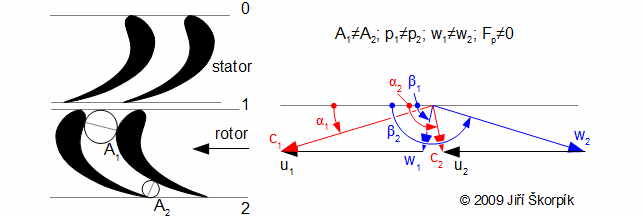6.280 An Example of the reaction stage (this stage is used also in Kaplan turbine(3)).
● 5 ●
● 12. Essential equations of turbomachines ●
(3)Remark
The Kaplan turbine is reaction turbine, it means, in front of the rotor is pressure higher than behind rotor (it can be easily identified by the fact that the blades forms convergent passages and for relative velocities w1<w2, p1>p2).

The axial force on rotor Fa of the axial reaction stages is higher (under overpressure of the working fluid), than for cases of the action stages. The working machine blade passage is usually made as reaction stage (can be used impulse stage theoretically also, but it is not used [1, p. 38-39]). The reaction stages has less power than the impulse stages under same conditions (tangential velocity; parameters of the working fluid), see article 19. Design of axials turbomachine stages.

The radial stages can be designed as the reaction stage also:The picture shows an example of an reaction radial stage.

The Euler equation can be use for "sparse" blade passages of wind turbines. The change of the velocity vector of the wind at flow through rotor is function the ratio u·c-1, which is called tip-speed ratio. The number of rotor blades is smaller with higher this ratio. It is means, the wind turbines with one blade have higher speed than three blades turbines (cheaper but higher noise), because one blade must transform the same energy as three blades:8.285 The force on blade of (u1=u2).
Momentum of wind is changed in all flow area formed by blades.
● 6 ●
● 12. Essential equations of turbomachines ●9.981 Change of tangential force on blades of an axial wind turbine rotor at change of blades number.
The derivation of the formula is shown in the Appendix 981.

## Relation between mean aerodynamic velocity and force F

A mean aerodynamic velocity wst is mean velocity between the inlet vector and the exit vector of the relative velocities w1, w2 of the blade passage. The Force on the blades from fluid stream F is perpendicular on the mean aerodynamic velocity for case of the incompressible flow:10.248 The definition of the mean aerodynamic velocity in the blade passage and its connection with the force on the blade (for case of the length of the blade dr).
Assumptions: incompressible flow, lossless flow (isentropic flow – subscript iz), axial stage (r1=r2). wst [m·s-1] mean aerodynamic velocity in blade passage; βst [rad] angle of mean aerodynamic velocity; ε [rad] angle of the force F. For incompressible flow is true w1a=w2a=wa, st. The derivation of this equation is shown in the Appendix 248.

## Force on blade and circulation of velocity

The force on blade inside an axial blade row can be calculated without pressures through circulation velocity on boundary of the control volume of the blade:

● 7 ●
● 12. Essential equations of turbomachines ●11.588 Force on blade and circulation of velocity.
Assumptions: blade length is elementary and the pitch of the blade s is constant. ρ [kg·m-3] density; Fl [N·m-1] force on airfoil about elementary length 1 m; Γ [m2·s-1] circulation of velocity. Γ circulation of velocity around the blade in blade row (on the curve of the control volume of blade ABCD on Figure 10); z number of blades; z·Γ circulation around z blades; ΓR1 circulation in front of the rotor blade row; ΓR2 circulation behind the rotor blade row. The derivation of this equation is shown in the Appendix 588.

From Equation 11(a) is evident, if be knew circulation in front of the rotor and behind the rotor then can be calculated the circulation around one the blade.

## Torque, power output

The forces on blades of the rotor from fluid stream develops a torque on its shaft. This torque is made up of tangential components these forces. The equation for torque of turbomachine stage is called Euler turbomachinery equation: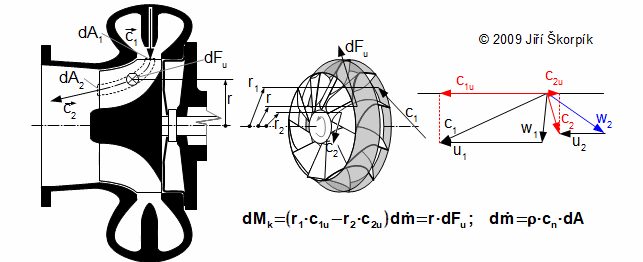12.275 The torque from an element of the working fluid inside the blade passage acting on the rotor (on picture is radial turbine with axial exit).
Mk [N·m] torque. dm· is mass flow rate through the area of the element dA1 of the blade passage and the exit area of the element dA2. This equation is derived for assumption stationary flow and assumption axially symmetric flow. The derivation of the Euler turbomachinery equation is shown in the Appendix 275.
● 8 ●
● 12. Essential equations of turbomachines ●

The torque is not function of the weight forces Fh (homogeneous accelerations field) neither the pressure forces Fp, because these forces have not any the sum forces components on tangential direction (respectively they annihilate between).

The power of the rotor can be calculated from the torque:13.585 The power transferred between the stream and the rotor (Euler turbomachinery equation).
P [W] power output.

## Discussion about Euler turbomachinery equation

For cases of a turbine stages is true dP>0, for cases of a working machine stages is true dP<0.

For cases of the axial stages (flow on a cylindrical surface, r1=r2=r) the Euler turbomachinery equation can be rearranged in this form: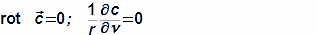14.586 Euler turbomachinery equation for the axial stage.

For case of short straight blades there is a change of the form of the velocity triangle along length the blade, therefore is approximately true:15.587 The power transferred between flow and the rotor with short the blades.

At higher speed rotation is transferred smaller the torque and opposite (for the same the power) according the Euler turbomachinery equation. Therefore the diameter of shafts of high-speed rotor of turbomachines are smaller than the diameter of shafts of low-speed rotor.

## Specific shaft work

The specific shaft work is a ratio between the power on rotor and mass flow rate of the working fluid (work of 1 kilogram of the working fluid during flow through the rotor blade row on tested radius r, which is transformed on torque of the machine shaft):16.284 Specific shaft work of the one turbomachine stage(4).
lu [J·kg-1] specific shaft work. lu is function of the velocities of stream in front of the rotor and the velocities of stream behind the rotor only, and is not function of processes inside the blades passage, according this equation. But the shape and the size of the velocity triangle on the exit of the blade passage is function these processes. The derivation of equation of the specific shaft work is shown in the Appendix 284.
● 9 ●
● 12. Essential equations of turbomachines ●
(4)Remark
The specific shaft work lu is not really the specific shaft work which leaving from the stage as the torque on the shaft. This work is decressing by a friction between the rotor and working fluid (so called rotor friction loss) and other losses of stage, more information in the article 14. Relation between specific shaft work and internal work of turbomachine stage).

## Discussion about specific shaft work

The quantities of the Equation 16 has different influence on the specific shaft work:

The absolute velocity is varied by all type of the turbomachine stages (there are the turbomachine stages, where c1=c2 e.g. [1, p. 40], but they do not used).

The relative velocity is not varied for a case of the impulse stages.

An influence of tangential velocity is significant for radial stages.

## Spiral passages inside turbomachinery

Working fluid flows on the spiral curve inside a spiral passage. The spiral passages can be classified to two types. The first type of the spiral passages are spiral casings(5), which are used as the enter/exit from/to the turbomachine of the working fluid. The next type of the spiral passages are vaneless diffuser(6), which are used on the turbocompressors and vaneless confusors(7), which are used on turbines.

(5)Spiral casings
For case of turbocompressors is the inlet to the spiral casing in radial direction and the outlet tangential direction, for a case of turbines is these opposite.
(6)Vaneless diffuser
The inlet and the outlet of the vaneless diffuser is in radial direction and working fluid is pressured inside (the pressure of the working fluid increasing here). The vaneless diffuser is usually formed by two annular wall, see bellow. On the vaneless diffuser continues a spiral casing.
(7)Vaneless confuser
The vaneless confuser is similar to the vaneless diffuser. Inside the vaneless confuser working fluid is expanded (the pressure of the working fluid decreasing here). A feeder for the vaneless confuser is a spiral casing.

Inside the spiral passages is presumed potential flow(8) at calculation or rot c=0.

The spiral passage has properties of a passage with constant flow area at incompressible flow ρ≈konst. –  it means, flow area of the spiral casing is changed with mass flow rate on tangential direction:

● 10 ●
● 12. Essential equations of turbomachines ●17.282 Simple equations for calculate the spiral casings.
a continuity equation. bs [m] width of the spiral passages; Aν [m2] flow area of the spiral passage under angle ν. The spiral passage must maintain constant pressure on perimeter of the impeller.
(8)Remark
Sometimes the spiral casing is computed with condition cu=const. (linear increase of flow area Aν). In this case is more simple the calculation, this spiral casing is smaller, but the losses are bigger, because the streamlines of working gas are plucked with the diameter and the flow is turbulent.
Design a spiral casing of low pressure radial fan with forward curved blades. Casing has rectangular passage section. Impeller perimeter is 65,82 mm, width of casing is 26,3 mm, tangential direction of absolute velocity at exhaust of impeller is 19,23 m·s-1 and flow of air is 100 m3·h-1. Discuss influence of width of casing on casing radius. The solution of this problem is shown in the Appendix 264.
Problem 5.264

For case of simple shape of the spiral casing (rectangular passage section, circular passage section) there is easy solving, but for case of intricate shape of the spiral casing is usually needed CFD computation.

For case of a vaneless diffuser or a confusor is the inlet flow area different from the outlet flow area: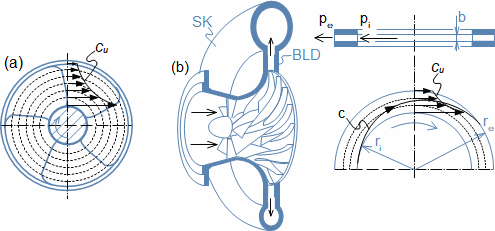18.391 Simplified section view through radial compressor with vaneless diffuser.
SK spiral casing; BLD vaneless diffuser; (a) general view; (b) cross section trough vaneless diffuser. In this case of the vaneless diffuser is formed by two annulus plates only.
● 11 ●
● 12. Essential equations of turbomachines ●
Derive equation of change of pressure inside a vaneless diffuser. Is there a change angle α (the angle between absolute velocity and tangential direction)? Calculate for case of incompressible flow. The solution of this problem is shown in the Appendix 407.
Problem 6.407

## Pressure and energy distribution inside turbomachine

Pressure distribution in the working fluid flow is influenced by change of flow area and curved of flow. The centrifugal force at curved flow develops increasing of pressure on outer perimeter so called transverse pressure gradient:19.673 Development of transverse pressure gradient inside curved passage.n normal of streamline; ρ' [m] radius of curvature of flow in tested point; dp/dn [Pa·m-1] pressure gradient. Derived for assumption of potential flow, 2D flow at negligible weight forces. This equation is called Euler n-equation. The derivation of Euler n-equation is shown in the Appendix 673.

The transverse pressure gradient is developed almost everywhere inside turbomachines:20.771 Examples development of the transverse pressure gradient inside turbomachines.(a) development of transverse pressure gradient in front of Kaplan turbine; (b) development of transverse pressure gradient inside spiral casing – exit pressure of working fluid pe is higher on outer perimeter, but its velocity is lower, therefore its total energy is the same through whole exit flow area.

The pressure gradient and kinetic energy gradient in front of and behind of a blade row for a calculation of velocity triangles are need to know only. Equation of the first law of thermodynamics for open system and Euler equation of fluid dynamics at potential flow be can use for calculation these gradients as well as gradients of other quantities of flow. The advantage this equation versus the Euler n-equation is that the gradients are in relation to direction of coordinate system and not to normals:

Calculate the pressure gradient in front of and behind of an axial water turbine. Assumptions: potential flow of ideal liquid. The solution of this problem is shown in the Appendix 736.
Problem 7.736
Calculate the pressure gradient inside the spiral casing from Problem 5. The solution of this problem is shown in the Appendix 725.
Problem 8.725
● 12 ●
● 12. Essential equations of turbomachines ●